# Window Calculations Overview

You can use window calculations to apply specific computations to measure values and compute running totals, percentages of totals, differences, and other analytical tasks.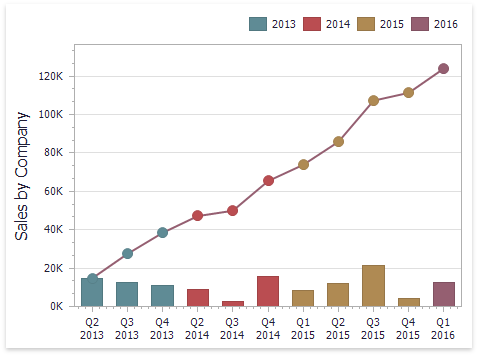You can apply window calculations to the measure values. The following calculation types are supported:

• Running Total - Allows you to calculate a cumulative total for a set of measure values.

Expression: RunningSum(Sum([Sales]))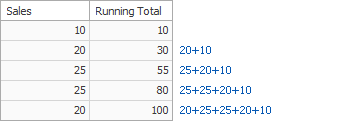• Moving Calculation - Allows you to apply a moving calculation, which uses neighboring values to calculate a total. Note that neighboring values are specified using offsets from the currently processed value.

Expression: WindowSum(Sum([Sales]), -1, 1)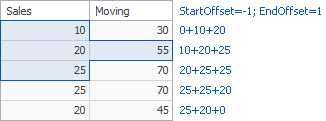• Difference - Allows you to compute differences between measure values.

Expression: Sum([Sales]) - Lookup(Sum([Sales]), -1)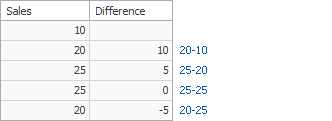• Percent of Total - Allows you to calculate a contribution of individual measure values to a total.

Expression: Sum([Sales])) / Total(Sum([Sales]))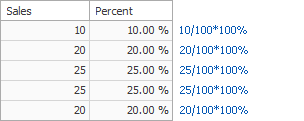• Rank - Allows you to rank values of the specified measure.

Expression: RankCompetition(Sum([Sales]), ‘asc’)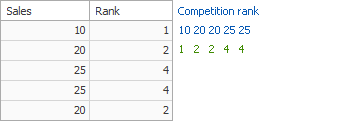Note that the computing of window calculations depends on two factors.

• The type of the dashboard item.

In this case, you need to specify a calculation direction that depends on the dashboard item type. For instance, the Pivot dashboard item provides the capability to apply calculations along its columns or rows.

• The set of dimensions that are used to calculate measure values.

In this case, a calculation direction depends on the dimensions’ order.

In both cases, measure values participating in a calculation fall into a specified window. For more details, see Window Definition.

For information on how to create a window calculation in the Dashboard Designer, see Creating Window Calculations.